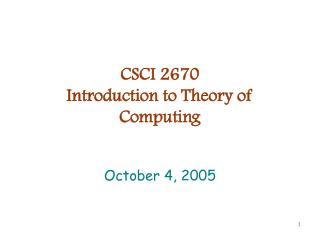# CSCI 2670 Introduction to Theory of Computing - PowerPoint PPT PresentationDownload PresentationCSCI 2670 Introduction to Theory of Computing

CSCI 2670 Introduction to Theory of ComputingDownload Presentation## CSCI 2670 Introduction to Theory of Computing

- - - - - - - - - - - - - - - - - - - - - - - - - - - E N D - - - - - - - - - - - - - - - - - - - - - - - - - - -
##### Presentation Transcript

1. CSCI 2670Introduction to Theory of Computing October 4, 2005

2. Agenda • Last week • Turing machines • This week • Sections 3.2 & 3.3 • Variants of Turing machines and the definition of algorithm October 4, 2005

3. Announcements • Homework due next Tuesday • 3.7, 3.15 b & c, 3.16 d • 3.7 and 3.15 are same in both texts • 3.16 d – Show the collection of Turing-recognizable languages is closed under the operation of intersection. • Quiz tomorrow • Turing machine definition, calculation, and design October 4, 2005

4. Group project 1 • Design a Turing machine to accept any string in {a,b}* after making a copy of it on the tape • The tape will start with w • After TM processes the string, the tape should read ww October 4, 2005

5. Group project 2 • Write a Turing machine that accepts the language {w  {a,b}* | |w| is even} October 4, 2005

6. Group project 3 • Write a Turing machine that accepts the language {anbm | nm and nm} October 4, 2005

7. Group project 4 • Write a Turing machine that accepts the language {anbman+m | n0 and m1} October 4, 2005

8. Group project 5 • Write a Turing machine that accepts the language {wwR | w{a,b}*} October 4, 2005

9. Group project 6 • Design a Turing machine that accepts the language {w{a,b}* | w has more a’s than b’s} October 4, 2005

10. Group project 1 • Design a Turing machine to accept any string in {a,b}* after making a copy of it on the tape • The tape will start with w • After TM processes the string, the tape should read ww October 4, 2005

11. TM design • Read first symbol • If a, replace with x, move to end and write x • If b, replace with y, move to end and write y • Move left to first x or y • Move left to next x or y • If x, replace with a and move right • If y, replace with b and move right October 4, 2005

12. TM design (cont.) • Read symbol at tape head • If x, replace with a and accept • If y, replace with b and accept • If neither, loop to beginning October 4, 2005

13. States • Start state q1 • Move right • Write a at end, write b at end, write x at end, write y at end • Move left to first x or y • Move left to second x or y & replace with a or b • Replace a or b with x or y October 4, 2005

14. qreject a → R b → R ~ → a,R a → L b → L ~ → L a → L b → L a,b,x,y → R a→ x,R ~ → x,R x → a,R y → b,R x → L y → L a → R b → R a→ x,R b→ y,R b→ y,R ~ → y,R a,b,x,y → R x → a,R y → b,R ~ → L ~ → b,R qaccept Copy machine October 4, 2005

15. Variants of Turing machines • Robustness of model • Varying the model does not change the power • Unlike finite automata (making finite automata nondeterministic changes the class of languages accepted) • Simple variant of TM model • Add “stay put” direction • Other variants • More tapes • Nondeterministic October 4, 2005

16. Multitape Turing machines • Same as standard Turing machine, but have several tapes • TM definition changes only in definition of δ δ : Q × Гk→ Q× Гk × {L,R}k October 4, 2005

17. Equivalence of machines Theorem: Every multitape Turing machine has an equivalent single tape Turing machine Proof method: construction October 4, 2005

18. 0 1 ~ ~ ~ ~ ~ ~ a a a ~ ~ ~ ~ ~ M a b ~ ~ ~ ~ ~ ~ # 0 1 # a a a # a b # ~ ~ S Equivalent machines October 4, 2005

19. Simulating k-tape behavior • Single tape start string is #w#~#...#~# • Each move proceeds as follows: • Start at leftmost slot • Scan right to (k+1)st # to find symbol at each virtual tape head • Make second pass making updates indicated by k-tape transition function • When a virtual head moves onto a #, shift string to right October 4, 2005

20. Corollary Corollary: A language is Turing-recognizable if and only if some multitape Turing machine recognizes it. October 4, 2005

21. Example • Using 2-tape Turing machine, write a copy machine • Copy tape 1 to tape 2 • Move tape 1 to beginning • Copy tape 1 to tape 2 • Accept October 4, 2005

22. qreject {a,~}→ {a,a},{R,R} {b,~}→ {b,b},{R,R} {a,~}→ {L,S} {b,~}→ {L,S} {a,~}→ {a,a},{R,R} {b,~}→ {b,b},{R,R} {a,~}→ {x,a},{R,R} {b,~}→ {y,b},{R,R} ~,~ → {L,S} {x,~} → {a,a},{R,R} {y,~} → {b,b},{R,R} {~,~} → {L,L} {~, ,~}→ {R,R} qaccept Copy machine October 4, 2005